Processing ......FreeComputerBooks.com Links to Free Computer, Mathematics, Technical Books all over the World

Computational and Inferential Thinking: The Foundations of Data Science
🌠 Top Free Mathematics Books - 100% Free or Open Source!
• Title: Computational and Inferential Thinking: The Foundations of Data Science
• Author(s) Ani Adhikari, John DeNero
• Publisher: Self Publishing (2021); eBook (Creative Commons Licensed)
• Hardcover/Paperback: N/A
• eBook: HTML/Jupyter Notebooks and PDF (631 pages)
• Language: English
• ISBN-10/ASIN: N/A
• ISBN-13: N/A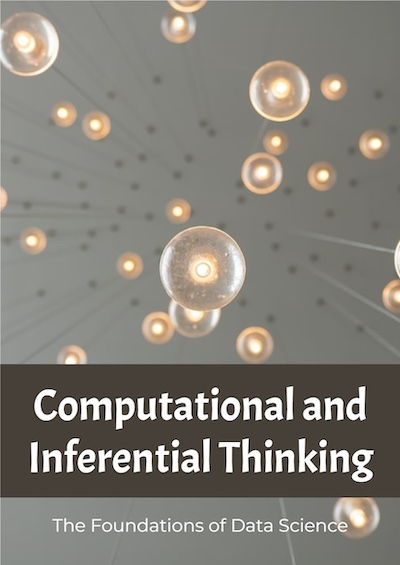Book Description

Data Science is about drawing useful conclusions from large and diverse data sets through exploration, prediction, and inference. Our primary tools for exploration are visualizations and descriptive statistics, for prediction are machine learning and optimization, and for inference are statistical tests and models.

Step by step, you'll learn how to leverage algorithmic thinking and the power of code, gain intuition about the power and limitations of current machine learning methods, and effectively apply them to real business problems.

• N/A
Reviews, Rating, and Recommendations: Related Book Categories: Read and Download Links:Similar Books:
•Statistical Inference: Algorithms, Evidence, and Data Science

A masterful guide to how the inferential bases of classical statistics can provide a principled disciplinary frame for the data science of the twenty-first century. Every aspiring data scientist should carefully study this book, use it as a reference.

•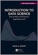Introduction to Data Science (Rafael A. Irizarry)

Introduces concepts and skills that can help tackling real-world data analysis challenges. Covers concepts from probability, statistical inference, linear regression, and machine learning. Helps developing skills such as R programming, data wrangling, etc.

•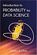Introduction to Probability for Data Science (Stanley Chan)

This book is an introductory textbook in undergraduate probability in the context of data science to emphasize the inseparability between data (computing) and probability (theory) in our time, with examples in both MATLAB and Python.

•Data Science at the Command Line, 2nd Ed. (Jeroen Janssens)

This hands-on guide demonstrates how the flexibility of the command line can help you become a more efficient and productive data scientist. Learn how to combine small, yet powerful, command-line tools to quickly obtain, scrub, explore, and model your data.

•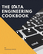Data Engineering Cookbook: The Plumbing of Data Science

This is a practical and comprehensive guide. You will learn the basics of data engineering. Then you will learn the technologies and frameworks required to build data pipelines to work with large datasets.

•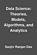Data Science: Theories, Models, Algorithms, and Analytics

It provides a bucket full of information regarding Data Science, covers a wide variety of sections by giving access to theories, data science algorithms, tools and analytics. You'll explore the right approach to best practices to guide you along the way.

•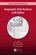Geographic Data Science with Python (Sergio Rey, et al.)

Provides the tools, the methods, and the theory to meet the challenges of contemporary data science applied to geographic problems and data, by using geographical and computational reasoning to unlock new insights hidden within data.

•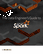The Data Engineer's Guide to Apache Spark (Databricks)

This book is for data engineers looking to leverage the immense growth of Apache Spark to build faster and more reliable data pipelines. It leverages Spark's amazing speed, scalability, simplicity, and versatility to build practical Big Data solutions.

•Python Data Science Handbook: Essential Tools (Jake VanderPlas)

Several resources exist for individual pieces of this data science stack, but only with the Python Data Science Handbook do you get them all - IPython, NumPy, Pandas, Matplotlib, Scikit-Learn, and other related tools.

•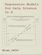Regression Models for Data Science in R (Brian Caffo)

The book gives a rigorous treatment of the elementary concepts of regression models from a practical perspective. The ideal reader for this book will be quantitatively literate and has a basic understanding of statistical concepts and R programming.

•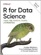R for Data Science: Visualize, Model, Transform, Tidy, Import

This book teaches you how to do data science with R: You'll learn how to get your data into R, get it into the most useful structure, transform it, visualize it and model it, how data science can help you work with the uncertainty and capture the opportunities.

•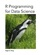R Programming for Data Science (Roger D. Peng)

This book is about the fundamentals of R programming. Get started with the basics of the language, learn how to manipulate datasets, how to write functions, and how to debug and optimize code. You will have a solid foundation on data science toolbox.

•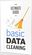The Ultimate Guide to Effective Data Cleaning

With this in-depth book, current and aspiring engineers will learn powerful real-world best practices for managing data big and small. Experts share their experiences and lessons learned for overcoming a variety of specific and often nagging challenges.

•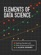Elements of Data Science (Allen B. Downey)

This book is an introduction to data science for people with no programming experience. The goal is to present a small, powerful subset of Python that allows you to do real work in data science as quickly as possible.

•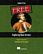Exploring Data Science (Nina Zumel, et al)

This book introduces readers to various areas in data science and explains which methodologies work best for each, with practical examples in R, Python, and other languages.

•Introduction to Data Science (Jeffrey Stanton)

This book provides non-technical readers with a gentle introduction to essential concepts and activities of data science. For more technical readers, the book provides explanations and code for a range of interesting applications using the open source R language for statistical computing and graphics.

•The Data Science Handbook: Advice and Insights

This book covers the essential exploratory techniques for summarizing data with R. These techniques are typically applied before formal modeling commences and can help inform the development of more complex statistical models.

•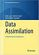Data Assimilation: A Mathematical Introduction (Kody Law, et al)

This book provides a systematic treatment of the mathematical underpinnings of work in data assimilation, covering both theoretical and computational approaches. Specifically the authors develop a unified mathematical framework of Bayesian formulation.

•School of Data Handbook

This textbook will provide the detail and background theory to support the Data Science courses and challenges. It will guide you through the key stages of a data project. These stages can be thought of as a pipeline, or a process.

Book Categories
 :All CategoriesTop Free BooksRecent BooksMiscellaneous BooksComputer EngineeringComputer LanguagesComputer ScienceData Science/DatabasesJava and Java EE (J2EE)Linux and UnixMathematicsMicrosoft and .NETMobile ComputingNetworking and CommunicationsSoftware EngineeringSpecial TopicsWeb Programming
Other Categories